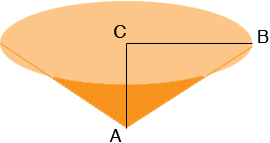SEARCH HOMEMath Central Quandaries & QueriesQuestion from Steven: I need to know how to find the weight of the contents of a silo that is 4 feet across and is funnel shaped at 60 degreesHi Steven,

I hope my diagram is correct.The distance from C to B is 2 feet and the angle CAB is 60 degrees.

The volume of a cone is 1/3 π r2 h here π is approximately 3.1416, r is the radius (2 feet) and h is the height which is the distance from A to C in the diagram. The tangent of the angle CAB is |CB|/|AC| and hence

tan(60) = 2/|AC|

and hence

h = |AC| = 2/tan(60) = 2/1.732 = 1.15 feet.

Hence the volume of the silo is

1/3 π 22 × 1.15 = 4.84 cubic feet.

To find the weight of the contents you need the density of the contents in pounds per cubic feet. Multiply the volume by the density to obtain the weight in pounds.

I hoe this helps,
PennyMath Central is supported by the University of Regina and The Pacific Institute for the Mathematical Sciences.